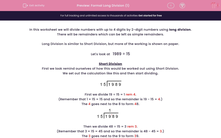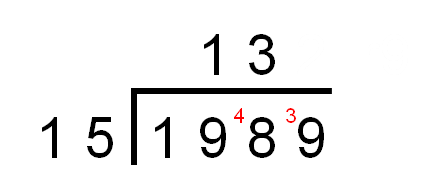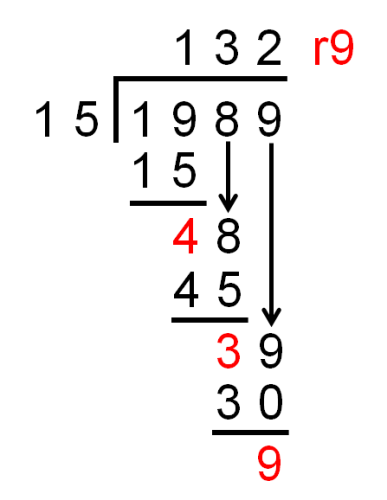# Formal Long Division (1)

In this worksheet, students must divide numbers up to 4 digits by a two-digit number using the formal written method of long division. There may be remainders.Key stage:  KS 2

Curriculum topic:   Maths and Numerical Reasoning

Curriculum subtopic:   Mixed Problems

Difficulty level:#### Worksheet Overview

In this worksheet we will divide numbers with up to 4 digits by 2-digit numbers using long division.

There will be remainders which can be left as simple remainders.

Long Division is similar to Short Division, but more of the working is shown on paper.

Let's look at   1989 ÷ 15

Short Division

First we look remind ourselves of how this would be worked out using Short Division.

We set out the calculation like this and then start dividing.First we divide 19 ÷ 15 = 1 rem 4.

(Remember that 1 × 15 = 15 and so the remainder is 19 - 15 = 4.)

The 4 goes next to the 8 to form 48.Then we divide 48 ÷ 15 = 3 rem 3.

(Remember that 3 × 15 = 45 and so the remainder is 48 - 45 = 3.)

The 3 goes next to the 9 to form 39.Lastly we divide 39 ÷ 15 = 2 rem 9.

(Remember that 2 × 15 = 30 and so the remainder is 39 - 30 = 9.)We have shown that    1989 ÷ 15 = 132 r9

Long Division

Now we look at the same division worked out using Long Division.

We start by setting out the calculation in the same way, like this, and then start dividing.First we divide 19 ÷ 15 = 1 rem 4.

(Remember that 1 × 15 = 15 and so the remainder is 19 - 15 = 4.)

In Long Division we actually set out the subtraction under the 19.In Short Division we put the 4 next to the 8 form 48.

In Long Division, we bring the 8 down next to the 4.Then we divide 48 ÷ 15 = 3 rem 3.

(Remember that 3 × 15 = 45 and so the remainder is 48 - 45 = 3.)

In Long Division, we bring the 9 down next to the 3.Lastly we divide 39 ÷ 15 = 2 rem 9.

(Remember that 2 × 15 = 30 and so the remainder is 39 - 30 = 9.)

The final remainder is 9.Compare this to short division.We have shown that    1989 ÷ 15 = 132 r9

Hint

With Long and Short Division, it helps to know the multiples of the number you are dividing by.

Here it helped to know the multiples of 15 are 15, 30, 45, 60, 75, 90 etc.

When dividing by 14, it helps to know the multiples of 14 are 14, 28, 42, 56, 70, 84 etc.

### What is EdPlace?

We're your National Curriculum aligned online education content provider helping each child succeed in English, maths and science from year 1 to GCSE. With an EdPlace account you’ll be able to track and measure progress, helping each child achieve their best. We build confidence and attainment by personalising each child’s learning at a level that suits them.

Get started